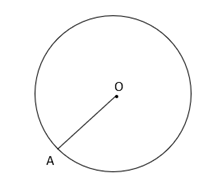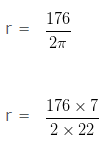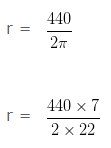A line segment having one end point on the circumference of the circle and other end point at the center of the circle is called the radius of the circle.Here, OA is the radius of the circle

Circumference of the circle

Circumference of a circle is the measure of the length of the circle.
Circumference of the circle = 2πr

Question 1

Find the radius of the circle whose circumference is 176 cm?

Explanation

Let, the radius of the given circle be r cm

As we know that,

Circumference of the circle = 2πr cm

Given,

Circumference of a circle = 176cm

2πr = 176 (from above)r = 28cm

Hence, the radius of the circle = 28cm

Question 2

Find the radius of the circle whose circumference is 440 cm?

Explanation

Let, the radius of the given circle be r cm

As we know that,

Circumference of the circle = 2πr cm

Given,

Circumference of a circle = 440cm

2πr = 440  (from above)r = 70cm

Hence, the radius of the circle = 70cm

### 2 thoughts on “Find Radius from Circumference”

1.2.Wow what a question❓
Practice question❓